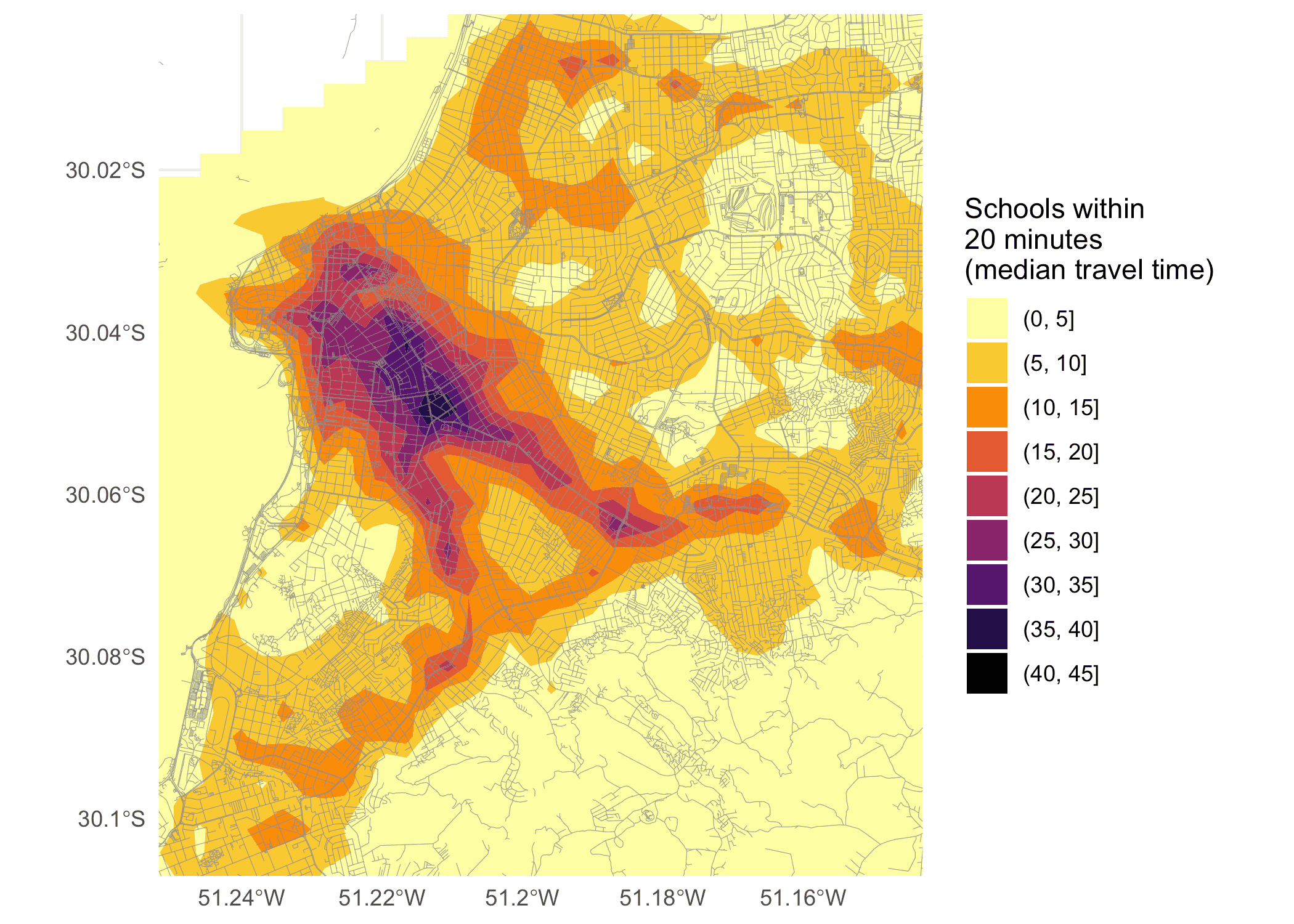# Calculating and visualizing Accessibility

#### 2021-07-02

Abstract

This vignette shows how to calculate and visualize accessibility in R using the r5r package.

## Introduction

Accessibility metrics measure the ease with which opportunities, such as jobs, can be reached by a traveler from a particular location (Levinson and et al. 2020). One of the simplest forms of accessibility metrics is the cumulative-opportunities, which counts all of the opportunities accessible from each location in less than a cutoff time. Using a travel time matrix and information on the number of opportunities available at each location, we can calculate and map accessibility. This vignette shows how to do that in R using the r5r package.

In this reproducible example, we will be using a sample data set for the city of Porto Alegre (Brazil) included in r5r. We can compute accessibility in 5 quick steps:

1. Increase Java memory and load libraries
2. Build routable transport network
3. Calculate travel times
4. Calculate Accessibility
5. Map Accessibility

## Increase Java memory and load libraries

Before we start, we need to increase the memory available to Java and load the packages used in this vignette

options(java.parameters = "-Xmx2G")

library(r5r)
library(sf)
library(data.table)
library(ggplot2)
library(akima)
library(dplyr)

## 1. Build routable transport network with setup_r5()

To build a routable transport network with r5r and load it into memory, the user needs to call setup_r5 with the path to the directory where OpenStreetMap and GTFS data are stored.

# system.file returns the directory with example data inside the r5r package
# set data path to directory containing your own data if not using the examples
data_path <- system.file("extdata/poa", package = "r5r")

r5r_core <- setup_r5(data_path, verbose = FALSE)

In this example, we will be calculating the number of schools accessible by public transport within a travel time of up to 20 minutes. The sample data provided contains information on the spatial distribution of schools in Porto Alegre in the points$schools. With the code below we compute the median number of schools accessible considering multiple travel time estimates departing every minute over a 60-minute time window, between 2pm and 3pm. # read all points in the city points <- fread(file.path(data_path, "poa_hexgrid.csv")) # routing inputs mode <- c("WALK", "TRANSIT") max_walk_dist <- 1000 # in meters travel_time_cutoff <- 21 # in minutes departure_datetime <- as.POSIXct("13-05-2019 14:00:00", format = "%d-%m-%Y %H:%M:%S") time_window <- 60 # in minutes percentiles <- 50 # calculate travel time matrix access <- accessibility(r5r_core, origins = points, destinations = points, mode = mode, opportunities_colname = "schools", decay_function = "step", cutoffs = travel_time_cutoff, departure_datetime = departure_datetime, max_walk_dist = max_walk_dist, time_window = time_window, percentiles = percentiles, verbose = FALSE) head(access) #> from_id percentile cutoff accessibility #> 1: 89a901291abffff 50 21 3 #> 2: 89a9012a3cfffff 50 21 0 #> 3: 89a901295b7ffff 50 21 6 #> 4: 89a901284a3ffff 50 21 1 #> 5: 89a9012809bffff 50 21 5 #> 6: 89a901285cfffff 50 21 3  ## 3. Map Accessibility The final step is mapping the accessibility results calculated earlier. The code below demonstrates how to do that, with some extra steps to produce a prettier map by doing a spatial interpolation of accessibility estimates. # interpolate estimates to get spatially smooth result access.interp <- access %>% inner_join(points, by=c('from_id'='id')) %>% with(interp(lon, lat, accessibility)) %>% with(cbind(acc=as.vector(z), # Column-major order x=rep(x, times=length(y)), y=rep(y, each=length(x)))) %>% as.data.frame() %>% na.omit() # find results' bounding box to crop the map bb_x <- c(min(access.interp$x), max(access.interp$x)) bb_y <- c(min(access.interp$y), max(access.interp$y)) # extract OSM network, to plot over map street_net <- street_network_to_sf(r5r_core) # plot ggplot(na.omit(access.interp)) + geom_contour_filled(aes(x=x, y=y, z=acc), alpha=.8) + geom_sf(data = street_net$edges, color = "gray55", size=0.1, alpha = 0.9) +
scale_fill_viridis_d(direction = -1, option = 'B') +
scale_x_continuous(expand=c(0,0)) +
scale_y_continuous(expand=c(0,0)) +
coord_sf(xlim = bb_x, ylim = bb_y) +
labs(fill = "Schools within\n20 minutes\n(median travel time)") +
theme_minimal() +
theme(axis.title = element_blank())If you have any suggestions or want to report an error, please visit the package GitHub page.